Home > INT1 > Chapter Ch5 > Lesson 5.3.3 > Problem5-120

5-120.
1. Describe the domain of each function or sequence below. 5-120 HW eTool (Desmos). Homework Help ✎

1. The function f(x) = 3x − 5.

2. The sequence t(n) = 3n − 5.

3. The function f(x) =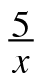.

4. The sequence t(n) =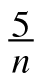.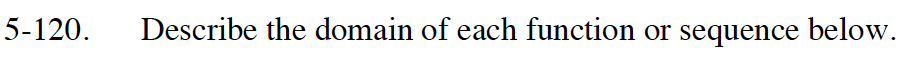The domain is any value of x that yields a real number value for y.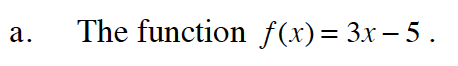Keep in mind that this is a sequence, not a function.

1, 2, 3...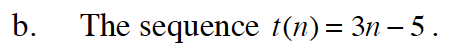Can 5 be divided by any number?

x cannot equal zero.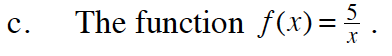Keep in mind that this is a sequence, not a function.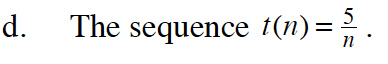Click on each graph in the eTool below to help you determine the domain of each function or sequence.
Click on the link at right for the full eTool version: Int1 5-120 HW eTool.